# Cohomotopy group

One of the generalizations of the one-dimensional cohomology group; a concept which is, in a certain sense, dual to that of homotopy group.

Let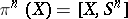be the set of homotopy classes of continuous mappings from a pointed spaceto the pointed sphere. The set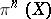does not always have a natural group structure. (This is the case only for, since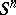is then a group.) The group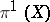is the same as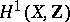.

Ifis a CW-complex of dimension at most, then a group structure can be defined onin the following way. Forone considers the mapping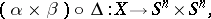where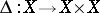is the diagonal mapping and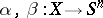are representatives of the classes. In view of the restriction on the dimension ofthere is a unique homotopy class of mappings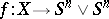(hereis a bouquet of pointed spheres) the composite of which with the natural inclusion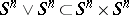is the same as the homotopy class of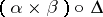. The homotopy class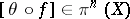of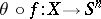, where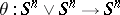is the folding mapping, is set equal to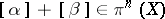. With respect to this operation the setis an Abelian group; therefore, the functoris often regarded as a functor defined only on the category of CW-complexes of dimension at most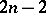, with values in the category of Abelian groups. For CW-complexesof dimension less than,. Thus, the functoris of interest in dimensions fromto, that is, in the so-called stable dimensions.

If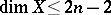, then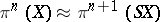, where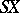is the suspension of. This isomorphism is given by the suspension functor: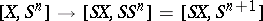. Ifis an arbitrary finite-dimensional CW-complex, then for sufficiently largethe set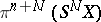has a group structure (for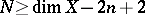one has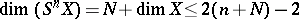). The groupwithis called the stable cohomotopy group of the CW-complex. The groups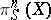are defined for all integer(and not merely positive integers). If one chooses fortwo points (one of which is distinguished), then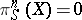for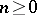,, and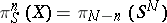are the stable homotopy groups of spheres for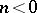.

If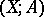is a pair of CW-complexes of dimension, then when, the relative cohomotopy group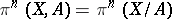is defined. One has the following exact sequence of Abelian groups: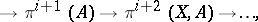extending indefinitely to the right; however, from some term onwards all groups are trivial: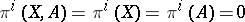when. This sequence extends to the left only as far as those values offor which. In this sequence the homomorphisms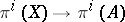and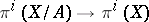are induced by the natural mappings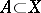and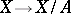. The homomorphism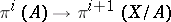is constructed as follows. For a classand a representative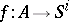of it, one chooses an extension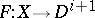ofdefined on the subspacewith values in. The mappinginduces a mapping, the homotopy class of which (an element of) is put in correspondence with the class.

If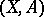is a pair of pointed CW-complexes of finite dimension, then there is the exact sequence of stable cohomotopy groups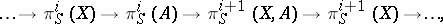extending indefinitely in both directions. This circumstance enables one to convert the stable cohomotopy groups into a generalized cohomology theory. For an arbitrary (non-pointed) finite-dimensional CW-complex, let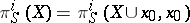, where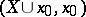is the pointed CW-complex obtained as the disjoint union ofwith a distinguished point. The functor, defined on the category of finite-dimensional CW-complexes, provides a generalized cohomology theory by settingThe value at a point of this theory is the same as the stable homotopy groups of spheres.

As for homotopy groups, the cohomotopy groups cannot be explicitly calculated even in the simplest cases, and this severely restricts the possibility of practical application of the above functors.